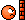# How can an atom reach less than absolute zero?

ok that makes a little more sense to me
so we have not gotten below 0K then ??

just read the link in the begining slowly and you will understand more

•hsdrop
Negative temperatures could be used to create heat engines — engines that convert heat energy to mechanical work, such as combustion engines — that are more than 100-percent efficient.

How???•hsdrop
DrClaude
Mentor
Negative temperatures could be used to create heat engines — engines that convert heat energy to mechanical work, such as combustion engines — that are more than 100-percent efficient.

How???You tell me. There is nothing magical about negative temperatures, and they do not lead to engines that are more efficient than the Carnot cycle.

In a sense, there is a lot of confusion ere that is simply based on an arbitrary choice for describing temperature. If instead of using ##T##, we used ##\tilde{\beta} \equiv -\beta = -1/k T##, then everything would make more intuitive sense. The coldest one could get to would be ##\tilde{\beta} = - \infty##, and that temperature would actually be impossible to reach (3rd law). Then, as ##\tilde{\beta}## increases, things go from cold to hot. Normal thermodynamics systems would be limited to negative temperatures, up to ##0_-##, while systems with a finite energy could have a positive ##\tilde{\beta}##, up to ##+ \infty##.

You tell me. There is nothing magical about negative temperatures, and they do not lead to engines that are more efficient than the Carnot cycle.
But just read the link in the begining they said so not me.

DrClaude
Mentor
But just read the link in the begining they said so not me.
This unusual advance could lead to new engines that could technically be more than 100 percent efficient, and shed light on mysteries such as dark energy, the mysterious substance that is apparently pulling our universe apart.What can I say? Don't learn science from news articles. This is 100% genuine cow manure.

f95toli
Gold Member
But just read the link in the begining they said so not me.
That part of the text was probably written by whoever is in charge of PR at their university. Press releases do -unfortunately- have a tendency to exaggerate the impact of results.

Personally I wouldn't even want to use the concept of temperature in this case. There are many non-equilibrium systems where the temperature is either ill defined or simply not meaningful.

What can I say? Don't learn science from news articles. This is 100% genuine cow manure.
Umm... That why i asked you guy.

•hsdrop
•Quantum Velocity
i think heat must flow from the positive to negative forHeat will flow from the hotter system to the colder one. That is the second law of thermodynamics. Actually, negative absolute temperature systems are hotter than positive ones, and are actually hotter than the infinite positive absolute temperature. You should take a look at the papers referenced in the link I gave below, it is pretty clear in there.

You have to think it this way: the definition for temperature can be given as:

##\displaystyle \left(\frac{\partial S}{\partial U}\right)_x=\frac{1}{T}##

But S is a concave function, this comes from thermodynamics (U is of course the internal energy). So to reach from positive to negative absolute temperatures, you must pass through a maximum of S:

##\displaystyle \left(\frac{\partial S}{\partial U}\right)_x=0\rightarrow T=\infty##.

With the system of spins is actually really easy to picture what happens. In the first place, you must have bounds in the energy so you can normalize the probability distributions.

If you place a system of spins in a magnetic field, the lowest energy state will be given with all the spins pointing in the same direction given by the external magnetic field. This is a zero entropy state, you have only one microstate for the given macrostate at T=0K. When you rise temperature, you will have some spins pointing in the direction of the external magnetic field, and others in the opposite direction, until you reach a maximum in entropy, a very disordered state, where there are many microstates compatible for the given macrostate at that temperature.

Now think of this situation, you start with the system in a positive absolute temperature state, close to T=0K, and suddenly you invert the external magnetic field: now the system will be trapped in a state of negative absolute temperature, with most spins pointing in the direction opposite to the external magnetic field. This is the population inversion that was mentioned before.

The thing is that the laws of thermodynamics and the usual statistical mechanics works in this negative absolute temperature states. You just change T by -T, and you see that the population of a given state will be:

##\displaystyle P_i \propto \exp{\left(\frac{E_i}{\kappa_B T}\right)}##,

so now the system tends to occupy the higher energy states, instead of the lower ones (which is the situation given by the sudden change in the direction of the external magnetic field).

Last edited:
•Quantum Velocity
thx alot Telemachus

•Telemachus